# EP1535389B1 - Motor drive control - Google Patents

## Info

Publication number
EP1535389B1
EP1535389B1 EP20030793876 EP03793876A EP1535389B1 EP 1535389 B1 EP1535389 B1 EP 1535389B1 EP 20030793876 EP20030793876 EP 20030793876 EP 03793876 A EP03793876 A EP 03793876A EP 1535389 B1 EP1535389 B1 EP 1535389B1
Authority
EP
European Patent Office
Prior art keywords
phases
inductance
phase
control
current
Prior art date
Legal status (The legal status is an assumption and is not a legal conclusion. Google has not performed a legal analysis and makes no representation as to the accuracy of the status listed.)
Active
Application number
EP20030793876
Other languages
German (de)
French (fr)
Other versions
EP1535389A1 (en
Inventor
Jeffrey Ronald Coles
Connel Williams
Current Assignee (The listed assignees may be inaccurate. Google has not performed a legal analysis and makes no representation or warranty as to the accuracy of the list.)
TRW Ltd
Original Assignee
TRW Ltd
Priority date (The priority date is an assumption and is not a legal conclusion. Google has not performed a legal analysis and makes no representation as to the accuracy of the date listed.)
Filing date
Publication date
Priority to GB0220401A priority Critical patent/GB0220401D0/en
Priority to GB0220401 priority
Application filed by TRW Ltd filed Critical TRW Ltd
Priority to PCT/GB2003/003817 priority patent/WO2004023639A1/en
Publication of EP1535389A1 publication Critical patent/EP1535389A1/en
Application granted granted Critical
Publication of EP1535389B1 publication Critical patent/EP1535389B1/en
Active legal-status Critical Current
Anticipated expiration legal-status Critical

• 244000171263 Ribes grossularia Species 0.000 claims description 108
• 230000000051 modifying Effects 0.000 claims description 24
• 230000001276 controlling effects Effects 0.000 claims description 4
• 230000001419 dependent Effects 0.000 claims 2
• 238000000034 methods Methods 0.000 description 31
• 238000010586 diagrams Methods 0.000 description 21
• 238000005259 measurements Methods 0.000 description 17
• 230000001939 inductive effects Effects 0.000 description 9
• 238000002347 injection Methods 0.000 description 8
• 239000007924 injections Substances 0.000 description 8
• 239000000203 mixtures Substances 0.000 description 7
• 230000005534 acoustic noise Effects 0.000 description 6
• 238000004804 winding Methods 0.000 description 6
• 230000000875 corresponding Effects 0.000 description 3
• 230000001934 delay Effects 0.000 description 3
• 230000000694 effects Effects 0.000 description 3
• 230000004907 flux Effects 0.000 description 3
• 230000001965 increased Effects 0.000 description 3
• 238000000691 measurement method Methods 0.000 description 3
• 241000287181 Sturnus vulgaris Species 0.000 description 2
• 239000011514 iron Substances 0.000 description 2
• 229910052742 iron Inorganic materials 0.000 description 2
• 230000000630 rising Effects 0.000 description 2
• 239000000243 solutions Substances 0.000 description 2
• 239000003570 air Substances 0.000 description 1
• 238000004458 analytical methods Methods 0.000 description 1
• 235000020127 ayran Nutrition 0.000 description 1
• 238000004364 calculation methods Methods 0.000 description 1
• 230000000295 complement Effects 0.000 description 1
• 229910052802 copper Inorganic materials 0.000 description 1
• 239000010949 copper Substances 0.000 description 1
• 238000001914 filtration Methods 0.000 description 1
• 238000010587 phase diagrams Methods 0.000 description 1
• 238000005070 sampling Methods 0.000 description 1
• 230000001360 synchronised Effects 0.000 description 1

## Images

•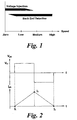•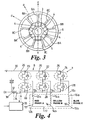•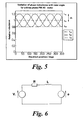•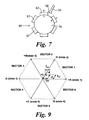•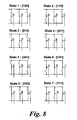•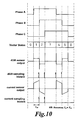•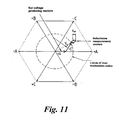•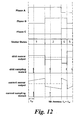•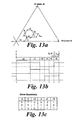•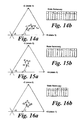•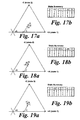•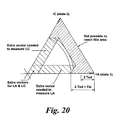•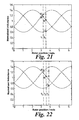•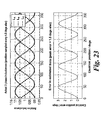•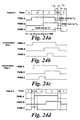•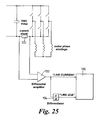## Classifications

• HELECTRICITY
• H02GENERATION; CONVERSION OR DISTRIBUTION OF ELECTRIC POWER
• H02PCONTROL OR REGULATION OF ELECTRIC MOTORS, ELECTRIC GENERATORS OR DYNAMO-ELECTRIC CONVERTERS; CONTROLLING TRANSFORMERS, REACTORS OR CHOKE COILS
• H02P6/00Arrangements for controlling synchronous motors or other dynamo-electric motors using electronic commutation dependent on the rotor position; Electronic commutators therefor
• H02P6/14Electronic commutators
• H02P6/16Circuit arrangements for detecting position
• H02P6/18Circuit arrangements for detecting position without separate position detecting elements
• H02P6/185Circuit arrangements for detecting position without separate position detecting elements using inductance sensing, e.g. pulse excitation

## Description

• This invention relates to electric motor control, and in particular to pulse width modulation (PWM) control of multiple phase brushless motors.
• In order to control an electric motor it is necessary to determine the position of the rotor as it rotates relative to the stator, so that the current through the motor windings can be controlled to produce the desired torque. This can be achieved using a dedicated position sensor, or by estimating the position from other parameters using a position sensorless control scheme.
• Position sensorless control schemes reduce system costs by replacing the position sensor that measures the rotor position with a position estimator algorithm in the controller. This algorithm determines the position of the rotor using knowledge of the applied phase or line-line voltages, the measured phase voltages, and a model of the motor drive system.
• There are many known techniques for implementing sensorless control, but they fall into two broad categories. The most established category is the back-emf detection algorithm, which is suitable for detecting the motor position from low speed to the maximum speed of the motor. Back-emf detection algorithms use a model of the motor fed with the known values of the applied voltages and measured voltages, which enables them to robustly determine the rotor back-emf, and hence the position of the rotor. However, these algorithms cannot detect the position down to zero speed because there is no back emf to detect at zero speed.
• The second category is the voltage-injection technique, which has emerged over recent years to enable the rotor position to be determined at low speeds and standstill. In voltage injection algorithms, a known voltage signal is superposed onto the normal applied phase voltages. The rate of change of current induced by this voltage signal is then measured, enabling the instantaneous inductance of that phase winding to be determined. By calculating the instantaneous inductance of all three phases, it is possible to detect the position of the rotor based on a simple model of inductance variation over position. These inductance variations will tend to be caused by either rotor saliency, localised saturation of the stator tooth tips due to the rotor field, or a combination of both. Since the injected voltage signal does not produce useful motive torque, it reduces the maximum "useful" voltage at the windings. To avoid compromising the power output of the motor, voltage injection techniques tend to be used in combination with a back-emf detection technique. The voltage injection technique provides position information from zero to low speed (where low speed is typically 10-20% of base speed), and the back-emf technique provides the position at low to high speeds, as shown in Figure 1.
• The principles underlying the voltage injection technique are as follows. A positive voltage is applied to one phase of the motor, and the resulting rate of change of the current is measured, A negative voltage is then applied and the rate of change of current is measured again. From the applied voltages and measured rates of change of current, it is possible to eliminate the unknown back-emf term and determine the instantaneous inductance of that phase. By measuring the instantaneous inductance of all three phases, the position of the rotor can be determined.
• An example of a known waveform is shown in Figure 2. This waveform is used to determine the inductance of a single phase. The phase current is sampled three times during the test pattern to determine the rising and falling rates of change of current. A different phase inductance is measured each time the test pattern is applied, so that after a period of typically a few ms all three of the phase inductances are known, and the position can be determined.
• Obviously with this technique, it is not possible to measure all three inductances simultaneously. However, each time a new inductance is measured, a new position is calculated. This calculation is based on the latest inductance value for each phase, so that although one phase will have a very recent measurement of inductance, the value for the other two phases will be somewhat older.
• This technique can work reasonably well and has some advantages such as the ability to determine position without prior knowledge of motor parameters and reasonably low computational requirements. However, there are some obvious disadvantages. One problem is that because the inductances are not sampled simultaneously, there is some error introduced into the position measurement. This error will be exacerbated if either the d- or q-axis inductances change rapidly with operating conditions, which could be the case with certain types of buried magnet motors. The delay between the measurements also introduces further time delays into the position response of the system. This reduces the dynamic capability of the drive, which can be a problem, particularly in a servo-drive system.
• The biggest problem with this approach is the generation of acoustic noise. Interrupting the PWM pattern every 1-2 ms produces a strong acoustic tone in the 250-500 Hz range. Practical demonstrations of such a system suggest that as it stands this noise would be unacceptable for most automotive applications.
• EP 0 856 937 discloses a motor position detection system in which the rate of change of current in the active stator coils is measured during the normal PWM cycle, and used to determine rotor positions.
• The present invention provides a drive system for a multi-phase brushless electric motor according to claim 1 and a method of controlling a multi-phase brushless electric motor according to claim 26.
• The control means is arranged to define at least one pair of test periods such that the phase voltages in one of the pair of test periods are opposite to those in the other of the pair of test periods and the control means is arranged to determine from the current measured in the pair of test periods the inductance of one of the phases. This means that the test voltages have zero net effect, and the algorithm for determining the output producing voltages does not need to be significantly modified.
• Normally, during any one PWM period, periods during which the voltages are reversed do not occur, since they will not add to the drive torque. Therefore it is normally necessary to add the test periods, as further periods within the PWM period in addition to the output producing periods when the voltages are producing the required output torque. The test periods will therefore normally occur between the output producing periods.
• The pair of test periods are both provided in the same PWM period.
• The control means may be arranged to measure the inductance of all of the phases in a single PWM period. In this case the control means is preferably arranged to switch the switching means to a number of conducting states during a number of output producing periods in a PWM period so as to produce a required motor output, and to a number of conducting states during said test periods, wherein the test periods are of sufficient length that the output producing periods and the test periods take up the whole of the PWM period.
• Alternatively the control means may be arranged to measure the inductance of only some of the phases in a single PWM period, for example for a motor having three phases, the control means may be arranged to measure the inductance of only two of the phases in a single PWM period. In this case, for any position of the motor two pairs can be selected from the three phases for which the inductance can be measured so that the PWM period includes a non-conducting state, and the control means is preferably arranged to measure the inductance of one of the pairs in one PWM period and the other pair in a subsequent PWM period. Preferably the control means is arranged to alternate inductance measurement between said pairs of phases in successive PWM periods.
• In a further alternative, the control means may be arranged to measure the inductance in only one of the phases in any one PWM period,
• Preferably the control means is arranged to vary the phases or phases for which the inductance is measured so that, over a number of PWM periods, the inductance of all of the phases is measured.
• Preferably the control means is arranged to provide a first PWM pattern in a first PWM period to allow inductance measurement of at least one phase and a second, different PWM pattern in a second PWM period to allow inductance measurement of at least one phase not measured in the first PWM period, and to produce at least one intermediate PWM pattern in a PWM period between the first and second periods, the intermediate pattern being intermediate in shape between the first and second patterns. This can help to reduce acoustic noise. The control means may need to be arranged not to measure the inductance of the phases in the intermediate PWM period.
• Preferably the control means is arranged to determine the position of the motor in each PWM period. Conveniently the control means may be arranged to determine the position of the motor on the basis of the last measured value of inductance for each of the phases.
• In some circumstances it is preferable for the control means to be arranged to determine the mean and peak values of the inductance of one of the phases as it oscillates during rotation of the motor, to measuring the instantaneous inductance of that phase, and to determine the position of the motor from the mean and peak and instantaneous values of the inductance. In this case it is preferable that, for all demanded voltages which can be produced from the same pair of primary voltages the inductance of the same two phases is measured, and when the demanded voltage changes so that a different pair of primary voltages are required and a different pair of inductances are measured, the latest measured values of the inductances of all of the phases are used to determine the mean and peak values of the inductance.
• Preferably the system further comprises a single current sensor arranged to measure the current in the phases during the test periods. In this case the control means is preferably arranged to measure the magnitude of the current in two phases in each PWM period using the current sensor thereby to determine the current in all of the phases. Furthermore the test periods are preferably each sufficiently long to allow the magnitude of the current in one of the phases to be measured by the current sensor.
• Alternatively, the system may include a plurality of current sensors, one for measuring the current in each of the phases.
• For systems in which only one phase inductance is measured in each PWM period, the control means is preferably arranged to define a group of demanded voltages which could be produced by switching the switching means between two conducting states and the time required in at least one of the conducting states is insufficient to allow measurement of the magnitude of the current, and for those demanded voltages to add further test periods of zero net voltage and sufficient length to enable the magnitude of the current in one of the phases to be measured. Preferably the further test periods are only added in some PWM periods. More preferably the extra test periods are only added when the inductance measuring test periods and the output producing periods together do not provide sufficient time in each of two conducting states within one PWM period to allow the magnitude of the current in one of the phases to be measured.
• Preferably the system includes a current sensor and a differentiator arranged to differentiate the output from the current sensor thereby to measure the phase inductances.
• Preferred embodiments of the present invention will now be described by way of example only with reference to the accompanying drawings in which:-
• Figure 1 shows the motor speeds at which two known methods of position sensing are used,
• Figure 2 shows a known voltage waveform used to measure the inductance of one phase of an electric motor,
• Figure 3 is a diagram of an electric motor according to the invention;
• Figure 4 is diagram of a drive circuit for a motor according to the invention;
• Figure 5 shows the variation of phase inductance with rotor angle in the motor of Figure 1;
• Figure 6 shows a simplified circuit model of one of the phases of the motor of Figure 1;
• Figure 7 shows an external magnet rotor according to a further embodiment of the invention;
• Figure 8 shows the eight states of the control circuit of the system of Figure 4;
• Figure 9 is a space vector diagram showing a pattern of test voltages used in the system of Figure 4;
• Figure 10 shows the phase voltages and sensor outputs resulting from the test voltage pattern of Figure 9
• Figure 11 is a space vector diagram showing a further pattern of test voltages used in the system of Figure 4;
• Figure 12 shows the phase voltages and sensor outputs resulting from the test voltage pattern of Figure 11 ;
• Figure 13a is a space vector diagram showing a further pattern of test voltages used for three-phase induction measurement in the system of Figure 4;
• Figure 13b shows the phase voltages resulting from the pattern of Figure 13a;
• Figure 13c is a table summarising the states resulting from the pattern of Figure 13a;
• Figure 14a is a space vector diagram showing a further pattern of test voltages used for two-phase induction measurement in the system of Figure 4, and Figure 14b is a summary of the resulting states;
• Figure 15a is a space vector diagram showing a further pattern of test voltages used for two-phase induction measurement in the system of Figure 4, and Figure 15b is a summary of the resulting states;
• Figure 16a is a space vector diagram showing a further pattern of test voltages used for two-phase induction measurement in the system of Figure 4, and Figure 16b is a summary of the resulting states;
• Figure 17a is a space vector diagram showing a further pattern of test voltages used for single-phase induction measurement in the system of Figure 4, and Figure 17b is a summary of the resulting states;
• Figure 18a is a space vector diagram showing a further pattern of test voltages used for single-phase induction measurement in the system of Figure 4, and Figure 18b is a summary of the resulting states;
• Figure 19a is a space vector diagram showing a further pattern of test voltages used for single-phase induction measurement in the system of Figure 4, and Figure 19b is a summary of the resulting states;
• Figure 20 shows areas of the space vector diagram where inductance and current sensing require the addition of further voltage vectors in the two-phase induction measurement arrangement;
• Figure 21 shows a method of measuring rotor position in the system of Figure 4;
• Figure 22 shows as further method of measuring rotor position in the system of Figure 4;
• Figure 23 shows the measured phase inductances and estimated errors in position measurements in the method of Figure 21;
• Figures 24a to 24d show how changes in the PWM pattern are controlled in the two-phase inductance measurement method switching between the patterns of Figure 14a and 15a;
• Figure 25 shows an alternative circuit for current an inductance measurement according to a further embodiment of the invention.
System Overview
• Referring to Figure 3 a three phase brushless motor 1 by way of example is provided which comprises a rotor 2 having for example six embedded magnets 4 within it which in this instance are arranged so as to provide six poles which alternate between north and south around the rotor. The rotor therefore defines three direct or d axes evenly spaced around the rotor and three quadrature or q axes interspaced between the d axes. The d axes are aligned with the magnetic poles of the magnets 4 where the lines of magnetic flux from the rotor are in the radial direction, and the q axes are spaced between the d axes where the lines of magnetic flux from the rotor are in the tangential direction.
• A stator 6 comprises a nine slot copper wound element having three groups of three teeth 8A, 8B, 8C each group of teeth having a common winding forming a respective phase. There are therefore three electrical cycles in each full rotation of the rotor, and the three teeth 8A, 8B, 8C in any phase are always in the same electrical position as each other.
• Referring to Figure 2, the three motor windings 12, 14, 16, generally designated as phases A, B and C, are connected in a star network. The phase windings are coiled around the stator teeth 8A, 8B, 8C respectively. One end 12a, 14a, 16a of each coil is connected to a respective terminal 12c, 14c, 16c. The other ends 12b, 14b, 16b, of the coils are connected together to form the star centre 17. A drive circuit comprises a three phase bridge 18. Each arm 20, 22, 24 of the bridge comprises a pair of switches in the form of a top transistor 26 and a bottom transistor 28 connected in series between a supply rail 30 and ground line 32. The motor windings 12, 14, 16 are each tapped off from between a respective complementary pair of transistors 26, 28. The transistors 26, 28 are turned on and off in a controlled manner by a controller 33 to provide pulse width modulation of the potential applied to each of the terminals 12c, 14c, 16c, thereby to control the potential difference applied across each of the windings 12, 14, 16 and hence also the current flowing through the windings. This in turn controls the strength and orientation of the magnetic field produced by the windings.
• A current measuring device in the form of a resistor 34 is provided in the ground line 32 between the motor 1 and ground so that the controller 33 can measure the total current flowing though all of the windings 12, 14, 16. In order to measure the current in each of the windings the total current has to be sampled at precise instants within the PWM period where the voltage applied to each terminal of the winding (and hence the conduction state of a particular phase) is known. A further sensor 36 which is a di/dt sensor arranged to measure rate of change of current is provided in the ground line 32 in series with the current sensor 34. The di/dt sensor 36 is used to measure the inductance of the phases, and hence the position of the rotor as will be described below.
• It will be appreciated from Figure 3 that the inductance of the rotor 2 varies with electrical position because the air gap between the stator iron and rotor back iron varies with position, resulting in a variation of stator flux reluctance with rotor position. The variation of inductance for each of the three phases A, B, C is shown in Figure 5.
• Referring to Figure 7, in a surface magnet rotor 60 the magnets 62 are mounted on the surface of the rotor core 64 and there is therefore very little or no rotor saliency to generate variation in inductance with rotor position. However, as one of the magnets 62 passes a stator tooth 66, localised magnetic saturation occurs in the stator tooth near the corners 70 of the magnets which alters the inductance of the stator windings. This therefore produces a regular variation in inductance with position which can be used in the same way as that arising in internal magnet rotors as described above to determine rotor position.
• Because there is a predictable and consistent variation of phase inductance with rotor position, the rotor position can be determined accurately from knowledge of the phase inductances. In a permanent magnet (PM) motor the inductance for a particular phase is generally highest when the q-axis is aligned with the phase, and lowest when the d-axis is aligned with the phase. Consequently, the inductance varies at twice the electrical frequency, and so the phase inductance equations will yield two possible rotor positions, 180 electrical degrees apart. The system needs to determine which of these two positions is the correct one. One suitable method is to determine the magnetic polarity at start-up by applying large positive and negative test currents, and comparing the effect of localised magnetic saturation on the resulting inductance measurements. Once this procedure has been carried out once, the magnetic polarity is known and the rotor position can always be determined uniquely.
• The model for the motor shown in Figure 5 assumes that phase inductance varies sinusoidally with position from its maximum value Lq to its minimum value Ld. Ld and Lq represent the phase inductances when the direct and quadrature rotor axes respectively are aligned with the stator axis of the phase being measured.
• The inductance variation shown in Figure 5 can be represented in complex co-ordinates as the sum of two contra-rotating vectors of length L0 and ΔL, where L0 is the average inductance and ΔL is half the peak-to-peak variation of inductance over one revolution, i.e. the amplitude of the variation in inductance. For L0 > ΔL, they are defined as: $L 0 = L q + L d 2 Δ ⁢ L = L q - L d 2$
• The inductance at a given rotor angle, L(θ), is the real part of the sum of the two complex contra-rotating vectors, i.e. $L θ = Re L 0 ⁢ e jθ - Δ ⁢ L ⁢ e - jθ$
• Which may be rearranged to yield $L θ = Re L 0 - Δ ⁢ L ⁢ e - 2 ⁢ jθ$
• The phase inductance measured at the three phases A, B and C is therefore $L A θ = Re L 0 - Δ ⁢ L ⁢ e - 2 ⁢ jθ L B θ = Re L 0 - Δ ⁢ L ⁢ e - 2 ⁢ j ⁢ θ - 2 ⁢ π / 3 L C θ = Re L 0 - Δ ⁢ L ⁢ e - 2 ⁢ j ⁢ θ - 4 ⁢ π / 3$
which can be re-written as: $L A θ = L 0 - Δ ⁢ L ⁢ cos 2 ⁢ θ L B θ = L 0 - Δ ⁢ L ⁢ cos 2 ⁢ θ - 2 ⁢ π / 3 L C θ = L 0 - Δ ⁢ L ⁢ cos 2 ⁢ θ - 4 ⁢ π / 3$
• This relationship is illustrated in Figure 5. If the three phase inductances are known, the three unknown variables, rotor angle θ, mean inductance L0 , and peak inductance ΔL can now be determined.
• L0 can be found from: $L 0 = L A θ + L B θ + L C θ 3$
• ΔL and θ can be found by first applying the Parkes transform to calculate the a and β frame inductances: $L α θ L β θ = 2 3 1 cos 2 ⁢ π / 3 cos 4 ⁢ π / 3 0 sin 2 ⁢ π / 3 sin 4 ⁢ π / 3 ⁢ L A θ L B θ L C θ$
• ΔL is then: $Δ ⁢ L = L α 2 θ + L β 2 θ$
and θ : $θ = - 1 2 ⁢ tan - 1 L β L α - π + nπ$
• Where n is an arbitrary integer which appears because the angle cannot be determined uniquely and which can be determined using the start-up routine described above.
• Determining Phase Inductance Online
• The phase inductance is determined by measuring the rates of change of current in the phase in response to a positive and a negative test voltage. This is a robust technique as it eliminates the back emf term from the system equations, which if not eliminated would be difficult to determine accurately and could lead to measurement errors.
• To determine the inductance of one phase the following steps are taken:
1. 1. Apply a positive voltage, +V, to the phase for sufficient time to determine the rising rate of change of current, di1 /dt.
2. 2. Apply a negative voltage, -V, to the phase for sufficient time to determine the falling rate of change of current, di2 /dt.
• Figure 6 shows a simplified circuit model of one phase of the drive, incorporating the applied voltage, V(t), the phase inductance, Lph , and the motor back-emf, e.
• When the positive test voltage is applied: $+ V - e = L ph ⁢ ⅆ i 1 ⅆ t + Ri 1$
• When the negative test voltage is applied: $- V - e = L ph ⁢ ⅆ i 2 ⅆ t + Ri 2$
• Since the average values of the currents i1 and i2 will be practically identical over the sample period, and since their difference (i1 - i2 ) is small, the resistance part can be neglected. Hence by subtracting (11) from (10) and rearranging, the phase inductance becomes: $L ph = ⅆ i 1 ⅆ t - ⅆ i 2 ⅆ t 2 ⁢ V$
• Hence, the instantaneous phase inductance is determined solely from the applied voltages and measured rates of change of current.
• The controller 33 is arranged to control the voltages applied to the phases A,B,C using pulse width modulation (PWM) to control the output torque of the motor as will now be described.
• Referring to Figure 8, each winding 2, 4, 6 in a three phase system can only be connected to either the supply rail 20 or the ground line 22 and there are therefore eight possible states of the control circuit. Using 1 to represent one of the phases being at positive voltage and 0 to represent a phase connected to ground, state 1 can be represented as  indicating phase A at 1, phase B at 0 and phase C at 0, State 2 is represented as , state 3 as , state 4 as , state 5 as , state 6 as , state 0 as  and state 7 as . Each of states 1 to 6 is a conducting state in which current flows through all of the windings 2, 4, 6, flowing in one direction through one of them and in the other direction through the other two. State 0 is a zero volt state in which all of the windings are connected to ground and state 7 is a zero volt state in which all the windings are connected to the supply rail.
• States 1, 2, 3, 4, 5 and 6 are herein also referred to as states +A, -C, + B, -A, + C and -B respectively, because they each represent the states in which the voltage applied across the windings is in a positive or negative direction for a respective one of the phases. For example in the + A state the A phase is connected to the supply rail and the other two phases are connected to the ground link, and in the -A state the connections are reversed.
• When the circuit is being controlled to produce pulse width modulation, each of the phases will normally be turned on and off once in each PWM period. The relative lengths of time that are taken up in each state will determine the magnitude and direction of the magnetic field produced in each winding, and hence the magnitude and direction of the total torque applied to the rotor. These lengths of time can be calculated by various modulation algorithms but in this embodiment a space vector modulation technique is used.
• Applying the Voltage Test Patterns
• Voltage test patterns that incorporate the positive and negative inductance measurement voltages across each of the phases are incorporated into the three-phase PWM waveform by inserting extra non-zero states into the applied PWM voltage pattern. This technique is conveniently described here using Space Vector Modulation (SVM), although any PWM modulation scheme could be adapted to implement the technique.
• Referring to Figure 9, in state vector modulation systems, the times in each PWM period spent in each of the states are represented as state vectors in a state vector modulation (SVM) diagram. In this type of diagram, single state vectors are those in the directions of the vectors S1 to S6, and the length of the vectors in each of these directions represents the amount of time in each PWM period spent in the respective state. This means that any desired voltage in the windings can be represented as a point on the diagram which corresponds to a voltage vector which represents the magnitude and direction of the voltage, and can be produced by a combination of state vectors s1, s2, etc. the length of which represent the time in each PWM period spent in that state.
• A Typical Test Pattern
• An example of test vectors, which represent the test voltages applied to the windings, is shown in Figure 9. Here two pairs of test vectors are applied which enables the inductance in two phases (A and C in this case) to be measured simultaneously, i.e. in the same PWM period. It can be seen that the pattern consists of a positive and negative phase A vector and a positive and negative phase C vector. It will be appreciated that, provided these vectors are the same length, i.e. the time spent in each of states 1 and 4 and in each of states 2 and 5 is the same, the net voltage from the test vectors will be zero.
• To measure the rate of change of current, a finite time is required for the sensors and associated circuitry to settle, and the reading to be taken. The minimum time required to measure the rate of change of phase current (di/dt) is herein defined as Tsd . Therefore each of the vectors must be of minimum length Tsd as illustrated in Figure 9.
• In order to keep Tsd as small as possible, a separate rate of change of current (di/dt) sensor 36 is used as described above. This enables the rate of change of current to be determined in a single reading, whereas a current sensor would have to be sampled twice to obtain this information.
• It can be seen that the test vectors shown in Figure 9 will also allow the phase currents to be measured using the single current sensor 34 in the ground return of the DC link. The minimum state time required to measure the current in a single sensor system is herein defined as Tsi , and to determine the currents in all three phases, the currents in at least two conducting states, which are not opposites of each other, need to be measured. Therefore providing there are two non-zero vectors from two phases of minimum length Tsi , the phase currents can be determined in a single PWM period.
• Figure 10 shows a typical PWM pattern for realising the state vectors of Figure 9. Typical outputs that would be expected for the link-return current sensor 34 and a link-return di/dt sensor 36 are also shown. All phase voltages go high-low-high. There are three periods of non-conducting states, two in state 0 and one in state 7, and four conducting states 1, 2, 4 and 5. During the conducting states the rate of change of current di/dt is substantially constant as the current rises at a constant rate. The current magnitude sensor di/dt sensor sample points are towards the end of the respective conducting state periods in which they are made to allow the current to settle after the switch between states.
• Combining the Test Pattern with the Applied Voltage Vector
• Figures 9 and 10 show test voltage vectors being applied for a modulation index of zero. For modulation indices greater than zero, the test vectors must be superposed onto the phase current-producing voltage demand vectors, which are calculated to produce the desired output torque from the motor. A typical example of this is shown in the space vector diagram of Figure 11. The demanded state vector v is made up of two primary state vectors + A and -C, and the four test period vectors are each of length Tsd Typical PWM patterns and sensor outputs for this case are shown in Figure 12.
• It can be seen from Figure 11 that superposing the test vectors onto the demand vectors reduces the time available in the PWM period for producing 'useful' voltage by 4Tsd.
• Analysis of Different Types of Test Vector Patterns
• The examples of Figures 11 and 12 show how it is possible to adapt the normal PWM waveform to measure two phase inductances simultaneously, i.e. in the same PWM period. However, the PWM waveform can be adapted to measure one, two or three inductances simultaneously, with different consequences for each.
• Test Vector Patterns to Measure Three Inductances Simultaneously
• Figure 13a shows the test vectors required to measure all three inductances simultaneously. The demand voltage is again made up of two vectors + A and -C, and there are six test voltage vectors each of length Tsd, the six test vectors comprising one each of the six primary vectors +A, -A, +B, -B, +C, -C and produce a net voltage of zero. Clearly, as all six non-zero vectors are needed, the available time for generating useful voltage is reduced by 6Tsd.
• Figure 13b shows a typical PWM pattern over one PWM period for realising these vectors, and Figure 13c shows the corresponding state summary. It can be seen that the PWM pattern has two unusual features. Firstly, it is not possible to apply zero-voltage state vectors, so the zero voltage states have to be replaced by increasing the size of each of the test vectors, each pair of opposite test vectors being kept of equal length so as to retain their net value of zero. Secondly, the operation of one of the phases must be the inverse of the other two. In other words, the state of the high-side transistors for two of the phases will go low-high-low in a PWM period, but the third phase they will go high-low-high. Although both of these features are unusual, they are physically possible and it should be possible to realise them in a practical system.
• Assuming the ideal case with zero dead time, it can be shown that the maximum modulation index mmax, defined herein as the ratio of the peak phase voltage fundamental to half of the DC link voltage, that can be applied whilst still measuring the instantaneous phase inductance in three phases is $m max = 2 3 ⁢ 1 - 6 ⁢ T sd T p$
where Tp is the PWM period.
• For a typical system where Tsd and Tsi are 10% of the PWM period Tp this would give a maximum theoretical modulation index of 0.46. Obviously in the real case the effects of interlock delays (dead times) would have to be accounted for which would reduce this number somewhat.
• The main advantage of the three-phase inductance measurement approach is that it enables the rotor position to be determined exactly each PWM period. The main drawbacks are that it severely limits the maximum modulation index, and that it produces a very unusual PWM pattern.
• Test Vector Patterns to Measure Two Inductances Simultaneously
• Two-phase measurement patterns (such as the one described above with reference to Figure 11) reduce the amount of time wasted on producing the test pattern to 4Tsd . Since only two phases are measured in a single PWM period, there are three different combinations of pairs of vectors that can be measured. Each combination will result in three different types of patterns, depending on which sector of the SVM vector space that the voltage demand lies. Some patterns are easier to realise than others.
• Figure 14a shows pattern I for sector 1 of the state vector diagram, and Figure 14b is the corresponding state summary. This comprises four non-zero state vectors and is the natural pattern for a particular sector as it is comprised only of the two principal state vectors for that sector and their inverses.
• Figure 14b shows pattern II. This comprises five non-zero state vectors and includes states from adjacent sectors. Unlike pattern I, zero-state vector seven cannot be used, but since zero-state vector zero can be realised, this should not present any real implementation problems.
• Figure 14c shows pattern III. This also comprises of five non-zero state vectors, but this time the only zero-state vector available is vector seven. The main problem with this pattern is that, as with the pattern for measuring three phase inductances, one phase of the resulting PWM waveform must go high-low-high, rather than low-high-low.
• A practical solution is to use patterns I and II only. By rapidly alternating between the two patterns, all three of the phase inductances can be determined. Table 1 shows which pattern should be selected for which phase measurement in each sector of the state vector diagram, the sectors being as shown in Figure 9. Table 1 Sector 1 2 3 4 5 6 Pattern I AC BC AB AC BC AB Pattern II BC AC AC AB AB BC
• The maximum modulation index, assuming zero interlock-delay, for two phase inductance measurements with any pattern is $m max = 2 3 ⁢ 1 - 4 ⁢ T sd T p$
• For the typical system described above where Tsd and Tsi , are 10% of the PWM period Tp this gives a maximum modulation index of 0.69.
• The two-inductance measurement technique significantly increases the maximum modulation index available. The PWM patterns produced are very similar to standard single current-sensor patterns, and are relatively easy to produce. By rapidly switching between patterns I and II, all three phase inductances can be determined. Furthermore, it may be possible to exploit the redundancy arising from the fact that one phase is measured twice to improve the accuracy of the position estimate.
• Test Vector Patterns to Measure One Inductance
• Figures 17a and 17b are a state vector diagram and state summary for measuring the inductance in only one phase, phase A in sector 1 of the vector space. Figures 18a and 18b are the corresponding diagrams for phase B and Figures 19a and 19b for state C. For phases A and C, the inductance can be measured without any restrictions to the PWM pattern. For phase B however, the pattern cannot incorporate the zero-state space vector seven, but again this should not present any major implementation problems. In general, for a given sector, the pattern required for measuring the inductance of the phase whose positive or negative vectors do not lie adjacent to that sector will not incorporate state vector seven.
• Since the test patterns only incorporate test vectors for one phase, it is no longer possible to guarantee that there will be enough time in the PWM waveform to measure two phase currents. For example if in the example of Figure 17a the -C vector were less than, Tsi , then only the current in phase A could be measured during any PWM period in which the inductance of phase A was being measured. Referring to Figures 18a and 19a, while the inductances of phases B and C are being measured, provided the vector + A is longer than Tsi then two phase currents can be measured. Hence, in order to carry out single current sensor current measurement successfully it is necessary in certain regions of the sector to add an extra test vector of length Tsi to incorporate sufficient time to measure one of the two phase currents required single current sensing. The regions where this is applicable are shown in Figure 20. It can be seen that for areas within Tsi of the + A or -A primary vector, extra test vectors will be needed when measuring the inductance of phase A, for areas within Tsi of the + B or -B primary vector, extra test vectors will be needed when measuring the inductance of phase B, and for areas within Tsi of the + C or -C primary vector, extra test vectors will be needed when measuring the inductance of phase C. For areas close to the centre of the phase diagram which are within Tsi of two state vectors, extra test vectors will be required to measure two of the inductances while maintaining full single sensor current measurement.
• Figure 20 also shows the limit of operation for test vector patterns that enable one phase inductance and two phase currents to be measured simultaneously. The maximum modulation index for zero interlock delays is therefore $m max = min 2 3 ⁢ 1 - 2 ⁢ T sd T p , 4 3 ⁢ 1 - T si + 2 ⁢ T sd T p$
where the function min{ x, y } returns the whichever is the smaller out of x and y.
• For the typical system described above where Tsd and Tsi are 10% of the PWM period Tp the maximum modulation index would be min{0.92, 0.93} = 0.93.
• The maximum modulation index could be increased either by not measuring the second phase current or by not measuring the inductance in the regions where an extra test vector would be needed. However, as the above result shows, the addition of extra test vector does not significantly reduce the maximum modulation index for practical implementations.
• The single-phase inductance measurement scheme gives the highest maximum modulation index, and so operates up to the highest speed. It generally yields slightly worse accuracy and dynamic response of the position estimate than with the two-phase approach, but providing sample rate is high, this is not particularly noticeable.
• Methods for Determining the Position
• Once the inductances of the phases are known, the rotor position can be determined by solving equations (5(a)-(c)). When all three phase-inductances are measured simultaneously, this process is straightforward. However, when only two or one of the inductances can be measured at a time, it is necessary to combine old and new inductance measurements to determine the position. The way in which these measurements are combined will affect the accuracy and dynamic characteristics of the position signal.
• Determining position from Three Simultaneous Inductance Measurements
• When all three phase inductances are measured simultaneously, the rotor position can be determined directly by solving the simultaneous equations (5(a)-(c)). This approach should give the position signal with the highest bandwidth and greatest accuracy.
• Determining position from Consecutive Inductance Measurements
• When the only one or two inductances are measured in each PWM period, the position can be estimated by using older inductance measurements for the phases that have not been measured at that sample-time. Figure 21 shows the situation where the inductances are measured in pairs. At time t2 the position can be determined by using the current value of the B and C phase inductances (LB2 and LC2) and the value of the phase A inductance from the previous sample (LA1) in the previous PWM period at time t1 . Since the old phase A inductance is being extrapolated forward in time, there will be a slight error in the computed position. However, if the angle travelled by the rotor, Δθ, during one sample time, Δt, is small the position error will be also be small.
• Figure 23 shows the simulated error in the computed rotor position obtained for this method when the inductances are measured in pairs. It can be seen that the position error varies sinusoidally at a rate four times the electrical frequency. It has been found empirically that the maximum error is approximately equal to one third of Δθ. It should be noted that since the estimate is based on a combination of current and previous measurements, the bandwidth of the position signal will be slightly less than for the three simultaneous readings due to the increased time delay.
• When only one inductance is measured at a time, the position is calculated from inductances measured at three different sample times, as shown in Figure 22. Empirically, the position error is slightly less than two thirds of Δθ, which is almost double the error that occurs when the inductances are measured in pairs.
• It can be seen that reducing the number of inductance measurements taken simultaneously will reduce the accuracy and bandwidth of the signal, but provided the measurements are taken often enough the reduction in signal quality will be small.
• Using the above techniques, it is possible to calculate the position signal at a much higher rate than is achieved with other known voltage injection techniques. Because of this, it is possible in some applications and implementations to apply filtering to the position signal to improve the signal quality. In particular, the position can be fed to an observer to give a more accurate representation.
• Potential Noise problems
• From the above discussion, it will be appreciated that the two-phase and single-phase inductance measurement techniques are a much more practical solution than the three-phase technique. However, in order to obtain an accurate high-bandwidth position signal using these approaches, it is necessary to swap rapidly between either two test vector patterns (patterns I and II) for the two-phase technique or three test vectors (phases A, B and C) for the single-phase technique.
• Figures 24a to 24d show typical PWM patterns for implementing the two-phase technique in sector 1. It can be seen that to get from pattern I as shown in Figure 24a which corresponds to Figure 14a, to pattern II as shown in figure 24d which corresponds to Figure 15a, the waveforms for phase A and B have to shift to the right by a fairly small distance (Tsd), and the waveform for phase C has to shift by a large distance to the left. In many circumstances, such discontinuous jumps would result in current discontinuities leading to an unacceptable level of acoustic noise. The jump is therefore executed over several PWM cycles. In this case a three-cycle update is used, the two intermediate patterns of Figures 24b and 24c being inserted between Pattern I and Pattern II. During PWM periods where the intermediate patterns are being used no inductance measurement is possible. However the frequency of inductance measurement will generally still be sufficient for most applications.
• It will be appreciated that the number of intermediate patterns needed will depend on the particular application, and in some cases it may need to be significantly greater than two. It will also be appreciated that the use of intermediate patterns may also be used for the single phase induction measurement.
• Alternative Technique - Determining L0 and ΔL
• An alternative technique for determining the rotor position is to use knowledge of the mean and peak inductance parameters, L0 and ΔL. If these parameters are known, it is possible to determine position from a single phase inductance measurements by solving the appropriate equation from equations 7(a)-(c). This has the advantage that rather than alternating between different phase inductance measurements every sample, the same phase can be used for inductance measurements over a complete sector. This can reduce the potential acoustic noise problems described above with reference to Figures 24a to 24d. Furthermore, if the inductances are measured in pairs, it may be possible to use the redundancy of the extra variable to correct any inaccuracies in the measurement.
• Position estimates made in this manner can be highly sensitive to errors in the values of ΔL and L0 . Factors such as motor temperature cause these values to drift during operation, so it is therefore preferable to estimate these values online and update them as often as possible. This can be done in the same manner as above, by taking successive inductance readings from separate phases, and using a combination of old and new measurements for the inductance of each phase to determine ΔL and L0 . One particularly convenient way to do this is to measure the inductances in pairs, with the same pair being measured over a whole sector, for example using patterns I from Figure 14a for the whole of sector 1. On the transition from one sector to the next, all three inductances are known within a maximum delay of one sample, so ΔL and L0 can be determined. The main criterion is that ΔL and L0 are updated often enough to follow any parameter drift. Updating each time the demanded voltage moves from one sector of the state vector diagram to the next will generally provide a fast enough update rate to achieve this.
• This technique may be less successful in motor topologies where the q-axis inductance varies significantly with the torque-producing current, as would be the case in some buried magnet motor designs. In this case, rapid variations of ΔL and L0 can be expected for rapid changes in the torque output of the machine. It is possible in some circumstances to use a model of the motor characteristics to compensate for this in a feed-forward manner. However, where this is not possible, the methods described above with reference to Figures 21 and 22 are generally more appropriate.
• Measuring the Rate of Change of Current
• A number of methods can be used to determine the rate of change of current (di/dt) in the phase windings to enable the inductance to be determined. One method is to put a current sensor in each phase winding, measure the current at one instant, measure it a short time later and compute the difference. This technique is also suitable for single current-sensor techniques. For example in the embodiment of Figure 4 the di/dt sensor can be deleted and the rate of change of current measured using multiple samples of the current sensor 34. The disadvantage of this technique is the time required for taking the two current measurements to determine di/dt. This can result in an impracticably large value of Tsd. Furthermore the quantisation limits of the analogue to digital converter (ADC) are likely to result in a very low-resolution di/dt signal.
• These problems are overcome in the system of Figure 4 by using the di/dt sensor 36 in the DC-link return path. This sensor only needs to be sampled once to determine the rate of change of current, which can significantly reduce Tsd , as well as improving the resolution of the di/dt signal.
• Referring to Figure 25, in an alternative arrangement the current sensor comprises a resistor 100 and a differential amplifier 102 the output of which varies with the voltage across the resistor 100 and therefore with the current flowing through it. An analogue differentiator 104 is arranged to differentiate the signal from the current sensor. The differentiated current measurement signal is then input to a separate A/D port on the digital controller 106, enabling the current and the rate of change of current to be determined simultaneously. Although differentiators can be noise sensitive, the signal would be measured between PWM edges, and would therefore be relatively insensitive to switching noise. Further noise reduction can be achieved by sampling the input over several PWM periods and taking the average value.
• The embodiments described can provide sensorless control over the entire speed range by combining back-emf observer techniques with the voltage injection technique described, in a similar manner to that shown in Figure 1. The embodiments described are specifically tailored for use in a single current-sensor system, which makes them ideal for automotive applications. The only additional hardware required over known systems is a single di/dt sensor for the DC-link return line, and an additional ADC port on the microcontroller. If an analogue differentiator is used to differentiate the signal from the current sensor, the number of physical sensors can be reduced further.
• It will be appreciated that the invention is, however, also applicable to systems with multiple current sensors, which have one current sensor in each phase of the motor. Such systems are more costly than single current sensor systems but are preferred in some applications.
• To overcome the acoustic noise problem, the system of the invention incorporates a suitable test voltage pattern into the PWM pattern, rather than using the approach of interrupting the PWM to inject a separate test pattern. Since the PWM is not interrupted, the acoustic noise resulting from the test patterns can be considerably lower than with known voltage injection systems.
• Furthermore, since the test voltage can, in most cases, be incorporated into every PWM cycle, the phase inductances can be determined much more frequently than with known techniques, which improves the dynamic characteristics of the position estimation signal. It also allows new techniques for calculating the position from the inductances.
• It will be appreciated that the invention is also applicable to other types of brushless machine including synchronous reluctance motors and inductance motors in which there is a degree of rotor saliency.
• ## Claims (26)

1. A drive system for a multi-phase brushless electric motor comprising a plurality of phases (A, B, C), the system comprising a drive circuit (18) including switch means (26, 28) arranged to varying the phase voltage applied across each of the phases, and control means (33) arranged to control the switch means so as to provide PWM control of the phase voltages to control the mechanical output of the motor, characterised in that: the control means (30) is further arranged to control the PWM voltage patterns so as to include at least one pair of test periods such that the phase voltages in one of the pair of test periods are opposite to those in the other of the pair of test periods; the control means is arranged to measure a rate of change of current in at least one of the phases during the test periods and to determine therefrom the inductance of at least one of the phases thereby to determine the rotational position of the motor; and the pair of test periods are both provided in the same PWM period.
2. A system according to any foregoing claim wherein the control means (30) is arranged to measure the inductance of all of the phases (A, B, C) in a single PWM period.
3. A system according to claim 2 wherein the control means (30) is arranged to switch the switching means (26, 28) to a number of conducting states during a number of output producing periods in a PWM period so as to produce a required motor output, and to a number of conducting states during said test periods, wherein the test periods are of sufficient length that the output producing periods and the test periods take up the whole of the PWM period.
4. A system according to claim 1 wherein the control means (30) is arranged to measure the inductance of only some of the phases (A, B, C) in a single PWM period.
5. A system according to claim 4 for a motor having three phases (A, B, C), wherein the control means (30) is arranged to measure the inductance of only two of the phases in a single PWM period.
6. A system according to claim 5 wherein for any position of the motor two pairs can be selected from the three phases (A, B, C) for which the inductance can be measured so that the PWM period includes a zero volt state, and the control means (30) is arranged to measure the inductance of one of the pairs in one PWM period and the other pair in a subsequent PWM period.
7. A system according to claim 6 wherein the control means (30) is arranged to alternate inductance measurement between said pairs of phases in successive PWM periods.
8. A system according to claim 1 wherein the control means (30) is arranged to measure the inductance in only one of the phases in any one PWM period.
9. A system according to any of claims 3 to 8 wherein the control means (30) is arranged to vary the phase or phases (A, B, C) for which the inductance is measured so that, over a number of PWM periods, the inductance of all of the phases is measured.
10. A system according to any of claims 8 to 11 wherein the control means (30) is arranged to provide a first PWM pattern in a first PWM period to allow inductance measurement of at least one phase and a second, different PWM pattern in a second PWM period to allow inductance measurement of at least one phase not measured in the first PWM period, and to produce at least one intermediate PWM pattern in a PWM period between the first and second periods, the intermediate pattern being intermediate in shape between the first and second patterns.
11. A system according to claim 10 wherein the control means (30) is arranged not to measure the inductance of the phases (A, B, C) in the intermediate PWM period.
12. A system according to any foregoing claim wherein the control means (30) is arranged to determine the position of the motor in each PWM period.
13. A system according to claim 12 wherein the control means (30) is arranged to determine the position of the motor on the basis of the last measured value of inductance for each of the phases.
14. A system according to claim 1 wherein the control means (30) is arranged to determine the mean and peak values of the inductance of one of the phases (A, B, C) as it oscillates during rotation of the motor, to measuring the instantaneous inductance of that phase, and to determine the position of the motor from the mean and peak and instantaneous values of the inductance.
15. A system according to claim 14 wherein, for all demanded voltages which can be produced from the same pair of primary voltages the inductance of the same two phases (A, B, C) is measured, and when the demanded voltage changes so that a different pair of primary voltages are required and a different pair of inductances are measured, the latest measured values of the inductances of all of the phases are used to determine the mean and peak values of the inductance.
16. A system according to any foregoing claim further comprising a single current sensor (100) arranged to measure the current in the phases during the test periods.
17. A system according to claim 16 wherein the control means (30) is arranged to measure the magnitude of the current in two phases in each PWM period using the current sensor (100) thereby to determine the current in all of the phases.
18. A system according to claim 17 wherein the test periods are each sufficiently long to allow the magnitude of the current in one of the phases to be measured by the current sensor (100).
19. A system according to claim 17 when dependent on claim 10 wherein the control means (30) is arranged to define a group of demanded voltages which could be produced by switching the switching means (26, 28) between two conducting states and the time required in at least one of the conducting states is insufficient to allow measurement of the magnitude of the current, and for those demanded voltages to add further test periods of zero net voltage and sufficient length to enable the magnitude of the current in one of the phases (A, B, C) to be measured.
20. A system according to claim 19 wherein the further test periods are only added in some pulse width modulation periods.
21. A system according to claim 20 wherein the extra test periods are only added when the inductance measuring test periods and the output producing periods together do not provide sufficient time in each of two conducting states within one PWM period to allow the magnitude of the current in one of the phases (A, B, C) to be measured.
22. A system according to any foregoing claim including a current sensor (102) and a differentiator (104) arranged to differentiate the output from the current sensor thereby to measure the phase inductances.
23. A system according to claim 22 when dependent on claim 18 wherein a single current sensor (102) is used for measurement of the magnitude of the current and inductance measuring.
24. A system according to any of claims 1 to 15 further comprising a plurality of current sensors for measuring the currents in the phases.
25. A system according to claim 24 wherein said current sensors comprise one current sensor for each of said phases.
26. A method of controlling a multi-phase brushless electric motor comprising a plurality of phases (A, B, C), the system comprising a drive circuit including switch means (26, 28) arranged to varying the phase voltage applied across each of the phases, the method comprising controlling the switch means so as to provide PWM control of the phase voltages to control the mechanical output of the motor, characterised in that the method further comprises: controlling the PWM voltage patterns so as to include at least one pair of test periods, such that the phase voltages in one of the pair of test periods are opposite to those in the other of the pair of test periods; measuring a rate of change of current in at least one of the phases during the test periods; and determining therefrom the inductance of at least one of the phases thereby to determine the rotational position of the motor wherein the pair of test periods are both provided in the same PWM period.
EP20030793876 2002-09-03 2003-09-03 Motor drive control Active EP1535389B1 (en)

## Priority Applications (3)

Application Number Priority Date Filing Date Title
GB0220401A GB0220401D0 (en) 2002-09-03 2002-09-03 Motor drive control
GB0220401 2002-09-03
PCT/GB2003/003817 WO2004023639A1 (en) 2002-09-03 2003-09-03 Motor drive control

## Publications (2)

Publication Number Publication Date
EP1535389A1 EP1535389A1 (en) 2005-06-01
EP1535389B1 true EP1535389B1 (en) 2009-12-30

# Family

## Family Applications (1)

Application Number Title Priority Date Filing Date
EP20030793876 Active EP1535389B1 (en) 2002-09-03 2003-09-03 Motor drive control

## Country Status (10)

US (1) US7015664B2 (en)
EP (1) EP1535389B1 (en)
JP (1) JP4614766B2 (en)
KR (1) KR101041050B1 (en)
CN (1) CN1326317C (en)
AU (1) AU2003264728A1 (en)
BR (1) BR0314004A (en)
DE (1) DE60330783D1 (en)
GB (1) GB0220401D0 (en)
WO (1) WO2004023639A1 (en)

## Families Citing this family (71)

* Cited by examiner, † Cited by third party
Publication number Priority date Publication date Assignee Title
CN100481708C (en) * 2001-09-29 2009-04-22 大金工业株式会社 Phase current detection method, inverter control method, motor control method, and apparatuses therefor
KR100631532B1 (en) 2004-09-10 2006-10-09 엘지전자 주식회사 Initial starting method for pmsm in using single current sensor
GB0422201D0 (en) 2004-10-07 2004-11-03 Trw Ltd Motor drive control
JP4592385B2 (en) * 2004-10-27 2010-12-01 株式会社東芝 Control device for synchronous machine
DE102005007995A1 (en) * 2005-02-22 2006-08-31 Robert Bosch Gmbh Rotor position determining method for e.g. electronically commutated motor, involves alternatively supplying pulse-width modulated voltage to stator lines such that lines are repeatedly energized with alternating polarities
KR100757060B1 (en) * 2005-04-01 2007-09-10 엘지전자 주식회사 A switched reluctance generator with enhanced generating efficiency at low speed
GB2428916B (en) * 2005-07-30 2009-04-15 Stored Energy Technology Ltd Synchronous motors
JP4497149B2 (en) * 2005-12-16 2010-07-07 パナソニック株式会社 Inverter device
GB0526274D0 (en) * 2005-12-23 2006-02-01 Trw Ltd Electric motor control
GB0526276D0 (en) * 2005-12-23 2006-02-01 Trw Ltd Electric motor control
US7462999B2 (en) * 2006-03-29 2008-12-09 Mitchell Electronics, Inc Brushless servo motor tester
EP1944860B9 (en) * 2007-01-12 2010-10-20 ABB Oy A method for sensorless estimation of rotor speed and position of a permanent magnet synchronous machine
DE102007004094A1 (en) * 2007-01-26 2008-08-07 Siemens Ag Method and device for controlling a pulse-width modulation controllable three-phase machine with a plurality of phase windings
US8138697B2 (en) * 2007-04-27 2012-03-20 International Rectifier Corporation Sensorless speed detection during zero vector
GB0709200D0 (en) * 2007-05-12 2007-06-20 Trw Ltd Current measuring apparatus for use with electric motors
KR100877599B1 (en) 2007-05-30 2009-01-09 영남대학교 산학협력단 Compensation method of Zero-Current-Clamping Effect in Pulsating Carrier-Signal Injection-Based Sensorless Drives
US8125170B2 (en) * 2007-06-16 2012-02-28 Board Of Regents, The University Of Texas System Techniques and apparatus for the measurement of mutual inductance in a switched reluctance machine
WO2009040965A1 (en) * 2007-09-27 2009-04-02 Mitsubishi Electric Corporation Controller of rotary electric machine
KR100915303B1 (en) * 2007-11-02 2009-09-03 영남대학교 산학협력단 Minimization of zero-current clamping effects in pulsating high frequency signal injection axis switching based sensorless permanent magnet motor drives
US7804264B2 (en) * 2008-02-02 2010-09-28 Science Bureau Inc. Commutation method and apparatus for a DC to AC inverter driving a three-phase asynchronous AC motor
TWI347737B (en) * 2008-02-27 2011-08-21 Prolific Technology Inc Method and pwm system of adjusting the width of pulses through collecting information of a three-phase current
CN101527537B (en) * 2008-03-07 2011-08-10 旺玖科技股份有限公司 Method for adjusting pulse width by capturing three-phase current information and system for modulating pulse width
FR2933550B1 (en) * 2008-07-01 2012-10-12 Schneider Toshiba Inverter Method for determining the inductions of a synchronous machine with permanent magnets
US8228013B2 (en) * 2008-09-10 2012-07-24 Rockwell Automation Technologies, Inc. Method and apparatus for automatically identifying electrical parameters in a sensor-less PMSM
DE102008043134A1 (en) * 2008-10-23 2010-04-29 Robert Bosch Gmbh DC motor and method of operating the DC motor
GB0822515D0 (en) * 2008-12-10 2009-01-14 Melexis Nv Operation of BLDC motors
JP5424814B2 (en) * 2009-05-21 2014-02-26 三菱電機株式会社 Permanent magnet type rotating electric machine
EP2442437B1 (en) * 2009-06-08 2019-12-18 Mitsubishi Electric Corporation Power converter
GB0920794D0 (en) 2009-11-27 2010-01-13 Trw Ltd Motor control circuitry
GB201004049D0 (en) 2010-03-11 2010-04-28 Trw Ltd Electric motor control
FR2962607B1 (en) * 2010-07-09 2012-09-07 Thales Sa Method for indexing a synchronous electric motor
GB201013957D0 (en) * 2010-08-20 2010-10-06 Trw Ltd Measurement circuit
US8531143B2 (en) * 2010-09-10 2013-09-10 Wisconsin Alumni Research Foundation Permanent magnet motor with stator-based saliency for position sensorless drive
WO2012157039A1 (en) * 2011-05-13 2012-11-22 株式会社日立製作所 Drive system for synchronous motor
ITBO20110340A1 (en) * 2011-06-13 2012-12-14 Spal Automotive Srl electric drive
GB201110039D0 (en) 2011-06-15 2011-07-27 Trw Ltd Measurement of motor rotor position or speed
US8912743B2 (en) * 2011-11-01 2014-12-16 Simmonds Precision Products, Inc. Apparatus and method of determining rotor position in a salient-type motor
GB2500014B (en) 2012-03-06 2016-09-21 Dyson Technology Ltd Sensorless control of a brushless permanent-magnet motor
US9088238B2 (en) * 2012-03-06 2015-07-21 Dyson Technology Limited Method of determining the rotor position of a permanent-magnet motor
US9515588B2 (en) 2012-03-06 2016-12-06 Dyson Technology Limited Sensorless control of a brushless permanent-magnet motor
JP5853096B2 (en) 2012-04-12 2016-02-09 株式会社日立製作所 Electric motor drive
DE112012006213T8 (en) * 2012-04-12 2015-01-15 Hitachi, Ltd. Drive device for a three-phase synchronous motor
GB201218674D0 (en) 2012-10-17 2012-11-28 Trw Ltd Control strategy for a motor of an electric assisted steering system
GB201220688D0 (en) * 2012-11-16 2013-01-02 Trw Ltd Improvements relating to electrical power assisted steering systems
CA2894141A1 (en) 2012-12-11 2014-06-19 Smart Wave Technologies Corp. Power management system for dispensers
GB201223174D0 (en) * 2012-12-21 2013-02-06 Trw Ltd Control of electric motors
US9093940B2 (en) * 2013-02-14 2015-07-28 Deere & Company Methods of determining initial position of a rotor and systems thereof
DE102013211017A1 (en) * 2013-06-13 2014-12-18 Robert Bosch Gmbh Control of an electric motor
US20160233804A1 (en) * 2013-10-22 2016-08-11 Mitsubishi Electric Corporation Motor control device
JP6429453B2 (en) * 2013-11-26 2018-11-28 キヤノン株式会社 Motor control apparatus and image forming apparatus
FR3016256B1 (en) * 2014-01-07 2016-01-22 Leroy Somer Moteurs Method for determining the polarity of a rotor pole of a rotating electric machine
DE102014201500A1 (en) * 2014-01-28 2015-07-30 Dr. Johannes Heidenhain Gmbh Method for diagnosing a converter
DE112014001983T5 (en) * 2014-02-18 2016-01-28 Mitsubishi Electric Corporation Engine control device and engine control method
JP5865930B2 (en) * 2014-03-07 2016-02-17 三菱電機株式会社 Motor control device
KR101628145B1 (en) * 2014-06-16 2016-06-09 현대자동차 주식회사 Method and system for controlling motor without sensor
JP2016021800A (en) * 2014-07-14 2016-02-04 株式会社リコー Position estimation device, motor drive control device, and position estimation method
JP6414109B2 (en) * 2016-03-18 2018-10-31 株式会社安川電機 AC motor rotation control device and AC motor rotation control method
EP3220535A1 (en) * 2016-03-18 2017-09-20 ABB Technology Oy Identification of synchronous inductances of a synchronous salient pole machine
US10116243B2 (en) * 2016-06-16 2018-10-30 Allegro Microsystems, Llc Determining motor position with complementary drive and detect and slight move
US10181810B2 (en) * 2016-06-16 2019-01-15 Allegro Microsystems, Llc Determining motor position with complementary driving and detection and current injection
US9831808B1 (en) * 2016-07-20 2017-11-28 Semiconductor Components Industries, Llc Rotor position sensing system for three phase motors and related methods
US9831809B1 (en) * 2016-07-20 2017-11-28 Semiconductor Components Industries, Llc Rotor position sensing system for three phase motors and related methods
DE102016214831A1 (en) * 2016-08-10 2018-02-15 Volkswagen Aktiengesellschaft Method and device for determining a direction of rotation of a rotating electrical machine, motor vehicle and computer program product
US9876452B1 (en) 2016-12-16 2018-01-23 Semiconductor Components Industries, Llc Rotor position sensing system for permanent magnet synchronous motors and related methods
WO2018202277A1 (en) * 2017-05-02 2018-11-08 Siemens Aktiengesellschaft Method of operation for an electric motor, and soft starter
KR101936261B1 (en) * 2017-09-21 2019-04-03 엘지전자 주식회사 Controlling apparatus for motor and method thereof
US10209137B1 (en) * 2017-12-22 2019-02-19 Kitty Hawk Corporation Measuring temperature in an electric motor
US10680483B2 (en) 2018-01-05 2020-06-09 Nidec Motor Corporation Motor with sectional housing
EP3591833A1 (en) * 2018-07-03 2020-01-08 Danfoss Power Electronics A/S Method for identifying the magnetic anisotropy of an electric rotary field machine
GB201813335D0 (en) * 2018-08-15 2018-09-26 Technelec Ltd Flux linkage to current observer for synchronous machines
GB2581187A (en) * 2019-02-07 2020-08-12 Stannah Stairlifts Ltd Electric motor control

## Family Cites Families (12)

* Cited by examiner, † Cited by third party
Publication number Priority date Publication date Assignee Title
NZ207431A (en) * 1984-03-08 1989-03-29 Fisher & Paykel Pulse with modulation controls current in dc motor
US5343129A (en) * 1990-06-18 1994-08-30 Papst Licensing Gmbh Drive circuit for a brushless direct-current motor
DE4039886C2 (en) * 1990-12-13 2002-05-08 Papst Licensing Gmbh & Co Kg Method and arrangement for commutation or for detecting the rotational position of the rotor of a brushless DC motor without external position sensors
JP3312520B2 (en) * 1995-01-24 2002-08-12 富士電機株式会社 Magnetic pole position detection device for motor
EP0748038B1 (en) * 1995-06-05 2002-08-21 Kollmorgen Corporation System and method for controlling brushless permanent magnet motors
US5821713A (en) * 1995-09-11 1998-10-13 Advanced Motion Controls, Inc. Commutation position detection system and method
JP3358504B2 (en) * 1997-07-30 2002-12-24 トヨタ自動車株式会社 Electrical angle detector for synchronous motor
DE19838227A1 (en) * 1998-08-22 2000-02-24 Frankl & Kirchner Procedure for determining position of rotor of synchronous motor has different voltages applied to motor phases and time from their application to attainment of given switch-off current wave measured
US6172498B1 (en) * 1998-09-29 2001-01-09 Rockwell Technologies, Llc Method and apparatus for rotor angle detection
CN1240180C (en) * 1999-09-20 2006-02-01 三菱电机株式会社 Pole-position detector of synchronous motor
US6555977B1 (en) * 2000-07-27 2003-04-29 Texas Instruments Incorporated Disk drive motor position detection using mutual inductance zero crossing
US6703805B2 (en) * 2002-04-12 2004-03-09 Mountain Engineering Ii, Inc. System for measuring the position of an electric motor

## Also Published As

Publication number Publication date
JP2005537774A (en) 2005-12-08
GB0220401D0 (en) 2002-10-09
CN1326317C (en) 2007-07-11
DE60330783D1 (en) 2010-02-11
US7015664B2 (en) 2006-03-21
EP1535389A1 (en) 2005-06-01
KR101041050B1 (en) 2011-06-17
WO2004023639A1 (en) 2004-03-18
CN1689220A (en) 2005-10-26
KR20050057148A (en) 2005-06-16
JP4614766B2 (en) 2011-01-19
AU2003264728A1 (en) 2004-03-29
US20050269982A1 (en) 2005-12-08
BR0314004A (en) 2005-07-19

## Similar Documents

Publication Publication Date Title
Ofori et al. A pulse-injection-based sensorless position estimation method for a switched reluctance machine over a wide speed range
US20160134216A1 (en) Circuits and Methods of Determining Position and Velocity of a Rotor
US8115428B2 (en) Electric motor control
JP2875529B2 (en) Drive device for sensorless brushless motor
US6121736A (en) Control apparatus for motor, and motor unit having the control apparatus
US6707265B2 (en) Rotor angle detecting apparatus for DC brushless motors
Acarnley et al. Review of position-sensorless operation of brushless permanent-magnet machines
Husain et al. Rotor position sensing in switched reluctance motor drives by measuring mutually induced voltages
EP2286509B1 (en) Position sensorless motor control
US8253360B2 (en) Vector controlled motor drive system implementing pulse width modulated (PWM) waveforms
JP5537565B2 (en) Control device for electric power equipment
US6661192B2 (en) Controlling a brushless DC motor
US6172498B1 (en) Method and apparatus for rotor angle detection
EP1944860B9 (en) A method for sensorless estimation of rotor speed and position of a permanent magnet synchronous machine
US9124206B2 (en) Method and arrangement for determining the position of a rotor in a brushless motor or in stepper motor
JP5150585B2 (en) Permanent magnet synchronous motor drive device
TWI451688B (en) Control circuit for electric motor and method for determining angular position and direction of the same
French et al. Direct torque control of permanent magnet drives
JP3755424B2 (en) AC motor drive control device
Bateman et al. Sensorless operation of an ultra-high-speed switched reluctance machine
EP1597816B1 (en) Method for effecting the power-optimal control of bldc motors
US7804266B2 (en) Motor control device
US5001405A (en) Position detection for a brushless DC motor
US6465975B1 (en) Method and system for controlling torque in permanent magnet brushless electric motors
US6850022B2 (en) Method and system for determining electronic commutation in brushless DC machines irrespective of the placement of rotor position sensors

## Legal Events

Date Code Title Description
AK Designated contracting states

Kind code of ref document: A1

Designated state(s): AT BE BG CH CY CZ DE DK EE ES FI FR GB GR HU IE IT LI LU MC NL PT RO SE SI SK TR

17P Request for examination filed

Effective date: 20050321

AX Request for extension of the european patent

Extension state: AL LT LV MK

RBV Designated contracting states (corrected)

Designated state(s): DE ES FR GB IT

DAX Request for extension of the european patent (deleted)
17Q First examination report despatched

Effective date: 20061019

AK Designated contracting states

Kind code of ref document: B1

Designated state(s): DE ES FR GB IT

REG Reference to a national code

Ref country code: GB

Ref legal event code: FG4D

REF Corresponds to:

Ref document number: 60330783

Country of ref document: DE

Date of ref document: 20100211

Kind code of ref document: P

PG25 Lapsed in a contracting state [announced via postgrant information from national office to epo]

Ref country code: ES

Free format text: LAPSE BECAUSE OF FAILURE TO SUBMIT A TRANSLATION OF THE DESCRIPTION OR TO PAY THE FEE WITHIN THE PRESCRIBED TIME-LIMIT

Effective date: 20100410

26N No opposition filed

Effective date: 20101001

REG Reference to a national code

Ref country code: GB

Ref legal event code: 732E

Free format text: REGISTERED BETWEEN 20130815 AND 20130821

REG Reference to a national code

Ref country code: FR

Ref legal event code: PLFP

Year of fee payment: 14

REG Reference to a national code

Ref country code: FR

Ref legal event code: PLFP

Year of fee payment: 15

REG Reference to a national code

Ref country code: FR

Ref legal event code: PLFP

Year of fee payment: 16

PGFP Annual fee paid to national office [announced from national office to epo]

Ref country code: IT

Payment date: 20180920

Year of fee payment: 16

PGFP Annual fee paid to national office [announced from national office to epo]

Ref country code: FR

Payment date: 20190925

Year of fee payment: 17

PGFP Annual fee paid to national office [announced from national office to epo]

Ref country code: GB

Payment date: 20190927

Year of fee payment: 17

PGFP Annual fee paid to national office [announced from national office to epo]

Ref country code: DE

Payment date: 20190927

Year of fee payment: 17

REG Reference to a national code

Ref country code: DE

Ref legal event code: R082

Ref document number: 60330783

Country of ref document: DE

Representative=s name: OESTREICHER, LUCAS, DIPL.-ING. (FH), DE